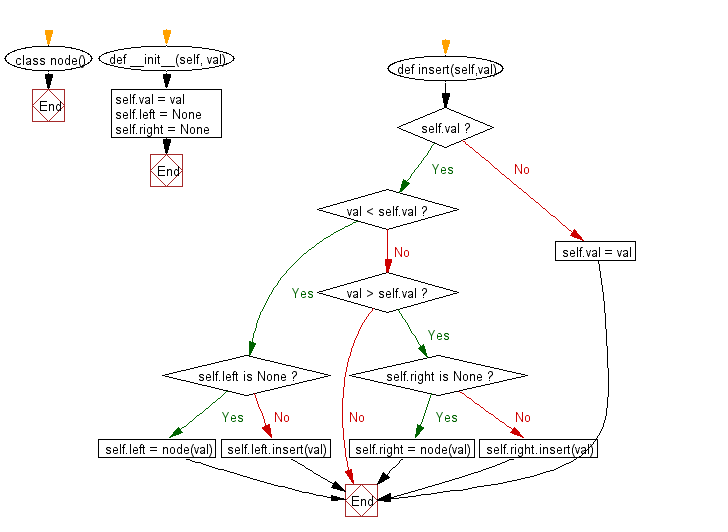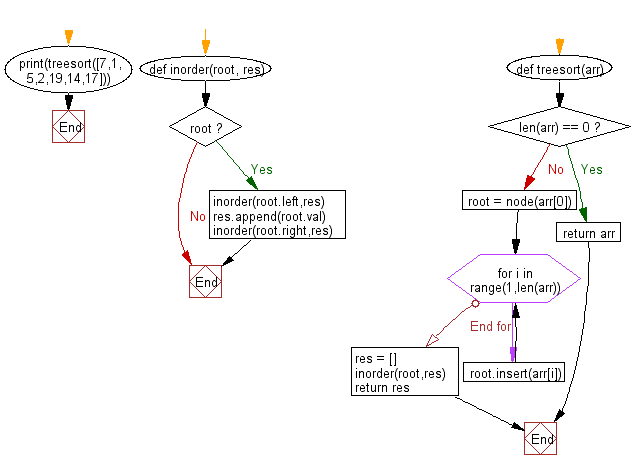﻿ Python: Sort a list of elements using Tree sort - w3resource# Python: Sort a list of elements using Tree sort

## Python Search and Sorting : Exercise-23 with Solution

Write a Python program to sort a list of elements using Tree sort.

Sample Solution:

Python Code:

``````# License https://bit.ly/2InTS3W
# Tree_sort algorithm
# Build a BST and in order traverse.
class node():
# BST data structure
def __init__(self, val):
self.val = val
self.left = None
self.right = None

def insert(self,val):
if self.val:
if val < self.val:
if self.left is None:
self.left = node(val)
else:
self.left.insert(val)
elif val > self.val:
if self.right is None:
self.right = node(val)
else:
self.right.insert(val)
else:
self.val = val

def inorder(root, res):
# Recursive travesal
if root:
inorder(root.left,res)
res.append(root.val)
inorder(root.right,res)

def treesort(arr):
# Build BST
if len(arr) == 0:
return arr
root = node(arr)
for i in range(1,len(arr)):
root.insert(arr[i])
# Traverse BST in order.
res = []
inorder(root,res)
return res

print(treesort([7,1,5,2,19,14,17]))
```
```

Sample Output:

```[1, 2, 5, 7, 14, 17, 19]
```

Flowchart:Python Code Editor:

What is the difficulty level of this exercise?

Test your Programming skills with w3resource's quiz.

﻿

## Python: Tips of the Day

The Zip() Function:

```>>> students = ('John', 'Mary', 'Mike')
>>> ages = (15, 17, 16)
>>> scores = (90, 88, 82, 17, 14)
>>> for student, age, score in zip(students, ages, scores):
...     print(f'{student}, age: {age}, score: {score}')
...
John, age: 15, score: 90
Mary, age: 17, score: 88
Mike, age: 16, score: 82
>>> zipped = zip(students, ages, scores)
>>> a, b, c = zip(*zipped)
>>> print(b)
(15, 17, 16)
```